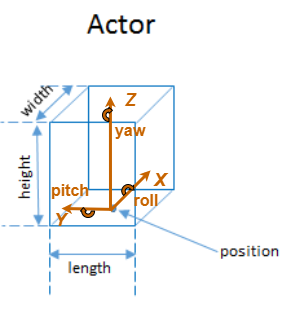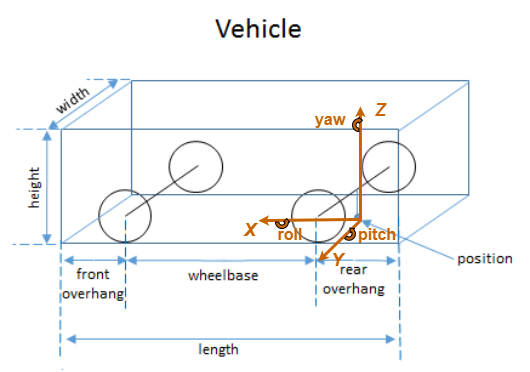Documentation

# actor

Add actor to driving scenario

## Syntax

``ac = actor(scenario)``
``ac = actor(scenario,Name,Value)``

## Description

````ac = actor(scenario)` adds an `Actor` object, `ac`, to the driving scenario, `scenario`. The actor has default property values.Actors are cuboids (box shapes) that represent objects in motion, such as cars, pedestrians, and bicycles. Actors can also represent stationary obstacles that can influence the motion of other actors, such as barriers. For more details about how actors are defined, see Actors and Vehicles.```

example

````ac = actor(scenario,Name,Value)` sets actor properties using one or more name-value pair arguments. For example, you can set the position, velocity, dimensions, and orientation of the actor.```

## Examples

collapse all

Create a driving scenario containing a curved road, two straight roads, and two actors: a car and a bicycle. Both actors move along the road for 60 seconds.

Create the driving scenario object.

`scenario = drivingScenario('SampleTime',0.1','StopTime',60);`

Create the curved road using road center points following the arc of a circle with an 800-meter radius. The arc starts at 0°, ends at 90°, and is sampled at 5° increments.

```angs = [0:5:90]'; R = 800; roadcenters = R*[cosd(angs) sind(angs) zeros(size(angs))]; roadwidth = 10; road(scenario,roadcenters,roadwidth);```

Add two straight roads with the default width, using road center points at each end.

```roadcenters = [700 0 0; 100 0 0]; road(scenario,roadcenters) roadcenters = [400 400 0; 0 0 0]; road(scenario,roadcenters)```

Get the road boundaries.

`rbdry = roadBoundaries(scenario);`

Add a car and a bicycle to the scenario. Position the car at the beginning of the first straight road.

`car = vehicle(scenario,'Position',[700 0 0],'Length',3,'Width',2,'Height',1.6);`

Position the bicycle farther down the road.

`bicycle = actor(scenario,'Position',[706 376 0]','Length',2,'Width',0.45,'Height',1.5);`

Plot the scenario.

```plot(scenario,'Centerline','on','RoadCenters','on'); title('Scenario');```Display the actor poses and profiles.

`poses = actorPoses(scenario)`
```poses=2×7 struct ActorID Position Velocity Roll Pitch Yaw AngularVelocity ```
`profiles = actorProfiles(scenario)`
```profiles=2×9 struct ActorID ClassID Length Width Height OriginOffset RCSPattern RCSAzimuthAngles RCSElevationAngles ```

## Input Arguments

collapse all

Driving scenario, specified as a `drivingScenario` object.

### Name-Value Pair Arguments

Specify optional comma-separated pairs of `Name,Value` arguments. `Name` is the argument name and `Value` is the corresponding value. `Name` must appear inside quotes. You can specify several name and value pair arguments in any order as `Name1,Value1,...,NameN,ValueN`.

Example: `actor('Length',0.24,'Width',0.45,'Height',1.7)` creates an actor with the dimensions of a pedestrian. Units are in meters.

Classification identifier of actor, specified as the comma-separated pair consisting of `'ClassID'` and a nonnegative integer.

Specify `ClassID` values to group together actors that have similar dimensions, radar cross-section (RCS) patterns, or other properties. As a best practice, before adding actors to a `drivingScenario` object, determine the actor classification scheme you want to use. Then, when creating the actors, specify the `ClassID` name-value pair to set classification identifiers according to the scheme.

Suppose you want to create a scenario containing these actors:

• Two cars, one of which is the ego vehicle

• A truck

• A bicycle

The code shows a sample classification scheme for this scenario, where `1` refers to cars, `2` refers to trucks, and `3` refers to bicycles. The cars have default vehicle properties. The truck and bicycle have the dimensions of a typical truck and bicycle, respectively.

```scenario = drivingScenario; ego = vehicle(scenario,'ClassID',1); car = vehicle(scenario,'ClassID',1); truck = vehicle(scenario,'ClassID',2,'Length',8.2,'Width',2.5,'Height',3.5); bicycle = actor(scenario,'ClassID',3,'Length',1.7,'Width',0.45,'Height',1.7);```

The default `ClassID` of `0` is reserved for an object of an unknown or unassigned class. If you plan to import `drivingScenario` objects into the Driving Scenario Designer app, do not leave the `ClassID` property of actors set to `0`. The app does not recognize a `ClassID` of `0` for actors and returns an error. Instead, set `ClassID` values of actors according to the actor classification scheme used in the app.

`ClassID`Class Name
`1`Car
`2`Truck
`3`Bicycle
`4`Pedestrian
`5`Barrier

Position of the actor center, specified as the comma-separated pair consisting of `'Position'` and an [x y z] real-valued vector.

The center of the actor is [L/2 W/2 b], where:

• L/2 is the midpoint of actor length L.

• W/2 is the midpoint of actor width W.

• b is the bottom of the cuboid.

Units are in meters.

Example: `[10;50;0]`

Velocity (v) of the actor center in the x-, y- and z-directions, specified as the comma-separated pair consisting of `'Velocity'` and a [vx vy vz] real-valued vector. The `'Position'` name-value pair specifies the actor center. Units are in meters per second.

Example: `[-4;7;10]`

Yaw angle of the actor, specified as the comma-separated pair consisting of `'Yaw'` and a real scalar. Yaw is the angle of rotation of the actor around the z-axis and is positive in the clockwise direction. Angle values are wrapped to the range [–180, 180]. Units are in degrees.

Example: `-0.4`

Pitch angle of the actor, specified as the comma-separated pair consisting of `'Pitch'` and a real scalar. Pitch is the angle of rotation of the actor around the y-axis and is positive in the clockwise direction. Angle values are wrapped to the range [–180, 180]. Units are in degrees.

Example: `5.8`

Roll angle of the actor, specified as the comma-separated pair consisting of `'Roll'` and a real scalar. Roll is the angle of rotation of the actor around the x-axis and is positive in the clockwise direction. Angle values are wrapped to the range [–180, 180]. Units are in degrees.

Example: `-10`

Angular velocity (ω) of the actor, in world coordinates, specified as the comma-separated pair consisting of `'AngularVelocity'` and a [ωx ωy ωz] real-valued vector. Units are in degrees per second.

Example: `[20 40 20]`

Length of the actor, specified as the comma-separated pair consisting of `'Length'` and a positive real scalar. Units are in meters.

Example: `5.5`

Width of the actor, specified as the comma-separated pair consisting of `'Width'` and a positive real scalar. Units are in meters.

Example: `3.0`

Height of the actor, specified as the comma-separated pair consisting of `'Height'` and a positive real scalar. Units are meters.

Example: `2.1`

Radar cross-section (RCS) pattern of actor, specified as the comma-separated pair consisting of `'RCSPattern'` and a Q-by-P real-valued matrix. RCS is a function of the azimuth and elevation angles, where:

• Q is the number of elevation angles specified by the `'RCSElevationAngles'` name-value pair.

• P is the number of azimuth angles specified by the `'RCSAzimuthAngles'` name-value pair.

Units are in decibels per square meter (dBsm).

Example: `5.8`

Azimuth angles of the actor's RCS pattern, specified as the comma-separated pair consisting of `'RCSAzimuthAngles'` and a P-element real-valued vector. P is the number of azimuth angles. Values are in the range [–180°, 180°].

Each element of `RCSAzimuthAngles` defines the azimuth angle of the corresponding column of the `'RCSPattern'` name-value pair. Units are in degrees.

Example: `[-90:90]`

Elevation angles of the actor's RCS pattern, specified as the comma-separated pair consisting of `'RCSElevationAngles'` and a Q-element real-valued vector. Q is the number of elevation angles. Values are in the range [–90°, 90°].

Each element of `RCSElevationAngles` defines the elevation angle of the corresponding row of the `RCSPattern` property. Units are in degrees.

Example: `[0:90]`

## Output Arguments

collapse all

Driving scenario actor, returned as an `Actor` object belonging to the driving scenario specified by `scenario`.

You can modify the `Actor` object by changing its property values. The property names correspond to the name-value pair arguments used to create the object. The only property that you cannot modify is `ActorID`, which is a positive integer indicating the unique, scenario-defined ID of the actor.

To specify or visualize actor motion, use these functions:

 `trajectory` Create actor or vehicle trajectory in driving scenario `chasePlot` Ego-centric projective perspective plot

To get information about actor characteristics, use these functions:

 `actorPoses` Positions, velocities, and orientations of actors in driving scenario `actorProfiles` Physical and radar characteristics of actors in driving scenario `targetOutlines` Outlines of targets viewed by actor `targetPoses` Target positions and orientations relative to ego vehicle `driving.scenario.targetsToEgo` Convert actor poses to ego vehicle coordinates

To get information about the roads and lanes that the actor is on, use these functions:

 `roadBoundaries` Get road boundaries `driving.scenario.roadBoundariesToEgo` Convert road boundaries to ego vehicle coordinates `currentLane` Get current lane of actor `laneBoundaries` Get lane boundaries of actor lane `laneMarkingVertices` Lane marking vertices and faces in driving scenario

collapse all

### Actors and Vehicles

In driving scenarios, an actor is a cuboid (box-shaped) object with a specific length, width, and height. Actors also have a radar cross-section (RCS) pattern, specified in dBsm, which you can refine by setting angular azimuth and elevation coordinates. An actor's position is defined as the center of its bottom face. This center point is used as the actor's rotational center and point of contact with the ground.A vehicle is a special kind of actor that moves on wheels. Vehicles have three extra properties that govern the placement of the front and rear axle.

• Wheelbase — Distance between the front and rear axles

• Front overhang — Distance between the front of the vehicle and the front axle.

• Rear overhang — Distance between the rear axle and the rear of the vehicle.

Unlike other types of actors, the vehicle's position is defined by the point on the ground that is below the center of its rear axle. This point corresponds to the vehicle's natural center of rotation.This table shows a list of common actors and their dimensions. To specify these values in `Actor` and `Vehicle` objects, set the corresponding properties shown.

Actor ClassificationActor ObjectActor Properties
`Length``Width``Height``FrontOverhang``RearOverhang``Wheelbase``RCSPattern`
Pedestrian`Actor`0.24 m0.45 m1.7 mN/AN/AN/A–8 dBsm
Car`Vehicle`4.7 m1.8 m1.4 m0.9 m1.0 m2.8 m10 dBsm
Motorcycle`Vehicle`2.2 m0.6 m1.5 m0.37 m0.32 m1.51 m0 dBsm